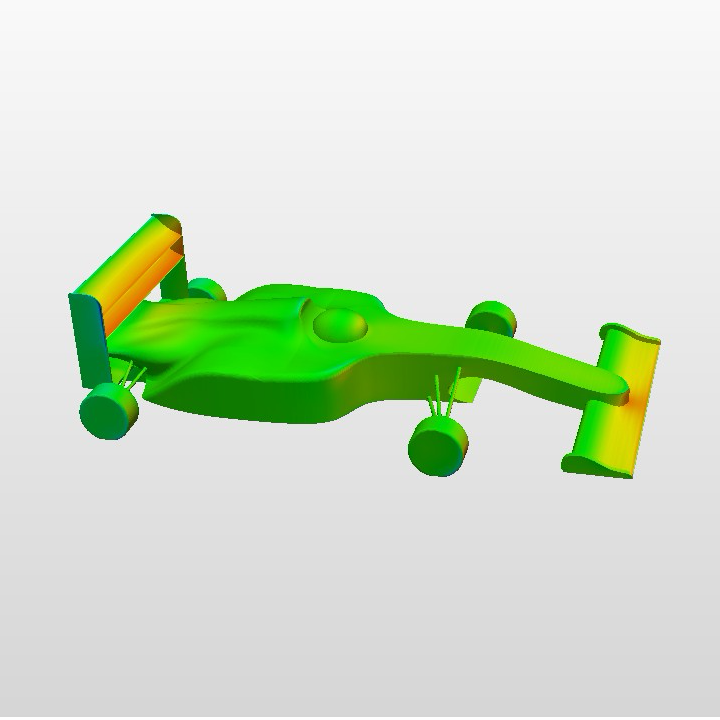# Introduction to Fluid Mechanics### Information

Bachelor in Aerospace Engineering. Course 2. 6 ETCS

### Cursos anteriores

- Calculus I & II
- Linear Algebra
- Physics I & II

### After this course

- Fundamental and applied knowledge of the laws that determine the fluid motion and their application to problems of interest in engineering.

### Syllabys

1. Introduction to Fluid Mechanics
1.1. Solids, liquids and gases.
1.2. The fluid as a continuum: Fluid particles.
1.3. Density, velocity and internal energy.
1.4. Local thermodynamic equilibrium.
1.5. Equations of state.

2. Flow kinematics
2.1 Coordinate systems
2.2 Eulerian and Lagrangian descriptions. Uniform flow. Steady flow. Stagnation points.
2.3 Trajectories. Paths. Fluid lines, Fluid surface, Fluid Volume.
2.4 Streamlines, stream surface and stream tubes
2.5 Material derivative. Acceleration
2.6 Circulation and vorticity.
2.7 Irrotational flow. Velocity Potential
2.8 Stream function
2.9 Local flow deformation. Strain-rate tensor
2.10 Convective flow
2.11 Reynolds transport theorem.

3. Conservation Laws
3.1. Continuity equation in integral form
3.2 Volume and surface forces
3.3 Stress tensor. Navier-Poisson law
3.4 Forces and moments on submerged bodies
3.5 Momentum equation in integral form
3.6 Angular momentum equation in integral form
3.7 Heat conduction
3.8 Energy equation in integral form. Formulation in terms of enthalpy and entropy.

4. Conservation equations in differential form: Navier-Stokes equations.
4.1 Continuity equation
4.2 Momentum equation
4.3 Energy equation. Internal energy and kinetic energy equations. Enthalpy and entropy equations.
4.4 Initial and boundary conditions
4.5 Bernoulli's equation.

5. Fluid statics
5.1 Equilibrium equations
5.2 Hydrostatics
5.3 Forces and moments on submerged bodies. Archimedes' Principle.
5.4 The standard atmosphere

6. Dimensional analysis
6.1 Dimensions of a physical magnitude
6.2 Physical quantities with independent dimensions
6.3 The Pi theorem
6.4 Nondimensionalization of the Navier-Stokes equations; Dimensionless numbers in Fluid Mechanics
6.5 Physical similarity. Partial similarity. Applications.7. Viscous flow
7.1 Uni-directional viscous flow in channels and pipes: Poiseuille and Couette flows
7.2 Uni-directional unsteady flows: Rayleigh's problem and Stokes' flow
7.3 Flows dominated by viscosity in ducts and channels of slowly varying cross section
7.4 The pipe entrance region
7.5 Introduction to hydrodynamic lubrication. The wedge effect.

### Bibliography

G. K. Batchelor. An Introduction to Fluid Dynamics. Cambridge University Press. 1967
L. D. Landau & E. M. Lifshitz. Fluid Mechanics. Pergamon Press. 1987
P. A. Lagerstrom. Laminar Flow Theory. Princeton University Press. 1996
G.F. Carrier, C.E. Pearson.. Ordinary Differential Equations. . SIAM (SIAM Classics in Applied Mathematics vol. 6). . 1991
T. M. Apostol. Calculus. John Wiley and Sons. 1969